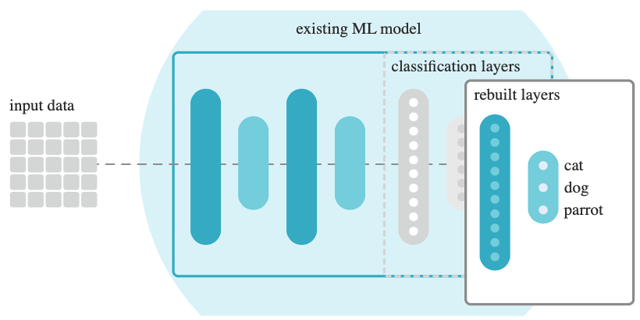## 探索MediaPipe自定义机器学习模型

MediaPipe支持人脸识别、目标检测、图像分类、人像分割、手势识别、文本分类、语音分类。每个模块都有对应的模型，但是原有模型可能比较大、推理耗时比较长，我们可以自定义模型来进行进行优化。

1、准备数据

1.1 原始数据

1.2 标注数据

2、简化模型

2.1 减少标签

2.2 剪裁边缘

2.3 模型复用

3、训练迭代

1、准备安装包

2、准备数据集

3、加载数据集

4、训练模型

5、验证模型

6、导出模型

## 一、训练准备

### 1、准备数据

#### 1.2 标注数据

``````# Requires Python >=3.7 <=3.9
pip install label-studio

# Start the server at http://localhost:8080
label-studio``````

### 2、简化模型

#### 2.3 模型复用## 二、目标检测训练

### 1、准备安装包

``````pip install --upgrade pip
pip install mediapipe-model-maker``````

``````import os
import tensorflow as tf
assert tf.__version__.startswith('2')

from mediapipe_model_maker import object_detector``````

### 2、准备数据集

``````train_dataset_path = "dogs/train"
validation_dataset_path = "dogs/validate"``````

### 3、加载数据集

``````train_data = object_detector.Dataset.from_pascal_voc_folder(
'dogs copy/train',
cache_dir="/tmp/od_data/train")

validate_data = object_detector.Dataset.from_pascal_voc_folder(
'dogs copy/validate',
cache_dir="/tmp/od_data/validatation")``````

### 4、训练模型

• batch_size=8
• learning_rate=0.3
• epochs=50

``````hparams = object_detector.HParams(batch_size=8, learning_rate=0.3, epochs=50, export_dir='exported_model')
options = object_detector.ObjectDetectorOptions(
supported_model=object_detector.SupportedModels.MOBILENET_V2,
hparams=hparams)
model = object_detector.ObjectDetector.create(
train_data=train_data,
validation_data=validate_data,
options=options)``````

### 5、验证模型

``````loss, coco_metrics = model.evaluate(validate_data, batch_size=4)
print(f"Validation loss: {loss}")
print(f"Validation coco metrics: {coco_metrics}")``````

### 6、导出模型

``````model.export_model('dogs.tflite')
!ls exported_model